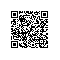# hdu 1811Rank of Tetris (并查集 + 拓扑排序)

/*
题意：这些信息可能有三种情况，分别是"A > B","A = B","A < B"，分别表示A的Rating高于B,等于B,小于B。

思路: 因为小于关系和大于关系可以转换一下位置！ 这里的问题就在与如何正确的处理相等的关系！
如果没有相等的关系，一个拓扑排序算法就可以搞定了！ 既然元素相等，那么我们取相等元素中的某一个
数来表示每一个数不是也行吗！？对，没错，用这个数来代替所有与之相等元素的数表示 '<'关系！ 也就是
转换成集合之间的关系的处理！ 将每一个相等的元素集合看成一个点，这个点的代表就是集合的父亲节点！

那么如何来得到这个数呢？并查集最适合不过了！我们将相等的元素放入集合中！
当 a<b时，通过getFather(a) < getFather(b)来处里a<b的关系，这里用邻接表进行处理！
*/
#include<iostream>
#include<cstring>
#include<vector>
#include<stack>
using namespace std;
int f;
int rank;
int n, m;
int getFather(int x){
return x==f[x] ? x : f[x]=getFather(f[x]);
}

int Union(int a, int b){
int fa=getFather(a), fb=getFather(b);
if(fa!=fb){
if(rank[fa]>rank[fb]){
f[fb]=fa;
rank[fa]+=rank[fb]+1;
}
else{
f[fa]=fb;
rank[fb]+=rank[fa]+1;
}
return 1;
}
return 0;
}

int in;
int A, B;
char ch;
vector<int>vx;
int conflict, uncertain;
int sum;

/*void topoSort(){
for(int j=1; j<=sum; ++j){
int p=0, cnt=0;
for(int i=1; i<=n; ++i)
if(f[i]==i && in[i]==0){//f[i]==i表明 i是这个相等集合的代表元素，也就是这个集合所有元素的父节点
p=i;
++cnt;
}
if(cnt==0){
conflict=1;
return;
}
if(cnt>1)
uncertain=1;
int len=vx[p].size();
for(int i=0; i<len; ++i)
--in[vx[p][i]];
in[p]=-1;
}
}*/

stack<int>ss;

void topoSort(){
for(int i=1; i<=n; ++i)
if(f[i]==i && in[i]==0)//f[i]==i表明 i是这个相等集合的代表元素，也就是这个集合所有元素的父节点
ss.push(i);
if(ss.size()==0 && sum)
conflict=1;
while(!ss.empty()){
int cnt=ss.size();
int p=ss.top();
--sum;//表示剩余多少个节点没有排序！
ss.pop();

if(cnt>1)
uncertain=1;
int len=vx[p].size();
for(int i=0; i<len; ++i)
if(--in[vx[p][i]]==0)
ss.push(vx[p][i]);
if(ss.size()==0 && sum)
conflict=1;
}

int main(){
while(cin>>n>>m){
for(int i=1; i<=n; ++i)
f[i]=i;
for(int i=1; i<=m; ++i){
scanf("%d %c %d", &A[i], &ch[i], &B[i]);
++A[i];
++B[i];
if(ch[i]=='=')
Union(A[i], B[i]);
}
sum=0;
for(int i=1; i<=n; ++i)
if(f[i]==i)  ++sum;
for(int i=1; i<=m; ++i){
int fa=getFather(A[i]), fb=getFather(B[i]);//将每一个相等的元素集合看成一个点，这个点的代表就是其父亲节点
if(ch[i]=='<'){
vx[fa].push_back(fb);
++in[fb];
}
else if(ch[i]=='>'){
vx[fb].push_back(fa);
++in[fa];
}
}

conflict=uncertain=0;
topoSort();
if(conflict)
cout<<"CONFLICT"<<endl;
else if(uncertain)
cout<<"UNCERTAIN"<<endl;
else cout<<"OK"<<endl;
for(int i=1; i<=n; ++i)
vx[i].clear();

memset(rank, 0, sizeof(int)*(n+1));
memset(in, 0, sizeof(int)*(n+1));
while(!ss.empty())
ss.pop();
}
}使用钉钉扫一扫加入圈子
+ 订阅